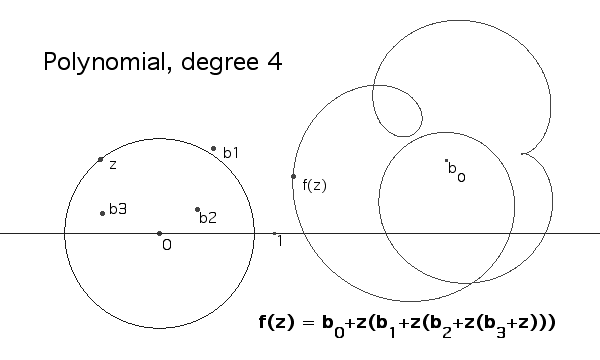# Complex Polynomials

In the study of complex polynomials one can learn something by looking at the image of a circle centred at the origin. Here we provide the source code for a dynamic spreadsheet that shows such an image, and allows the user to explore its appearance for various input values. In each figure there is a circle Cr with center 0 and radius r. Also shown are points b0, b1, b2, and so on, each of which represents a complex number used to define the polynomial f given by

f(z) = b0 + b1z + b2z2 + ... + bnzn

In each example given here (n = 2, 3, and 4) the polynomial is monic, that is, bn = 1. The values of r, and bi (for i = 0, .. n-1) may be controlled by the user.

The idea is to understand f(Cr), the locus of f(w) for all w on Cr. The Cabri figure shows this locus and as well, a particular point "z" which may be Animated to move around Cr. As it does so, the point "f(z)" traverses the curve f(Cr).

It is interesting to see the global changes in f(Cr) as r and bi (for i = 0, .. n-1) are changed. For example, for relatively small values of r, changes in the number of times f(z) goes around b b0 depending on the magnitudes of the various coefficients of f. For example, when the degree of f is 3, if b1 and b2 are small enough, f(z) winds three times around b0, but if b1 is moved far enough outside the circle of radius 1, f(z) winds only two times around b0. As the values of the coefficients of f and the radius r are modified, one might see loops getting larger or smaller. When loops shrink, a cusp might appear and then cease to exist as the curve becomes smooth again, leaving little or no evidence of its existence except perhaps for a hint of a dimple.

When z is animated on Cr it is also interesting to watch the micro behaviour of f(z) as it moves along the locus, in some parts of the curve moving faster and other parts moving slower, and even sometimes coming to a momentary stop and reversing its direction at a cusp.

Explorers might wish to consider these questions:

• What happens to f(Cr) when r is small. For example, does f(Cr) necessarily wind around the point b0? If so, how many times? How does this depend on the relative sizes of b1, b2, b3 and so on?
• Can you always select a value of r so that the origin is completely outside the locus f(Cr)?
• What happens to f(Cr) when r gets large? Does it necessarily wind around the origin? How many times ?
• As the size of r varies from small to large. what happens to the curve f(Cr)?
• Is it necessarily the case that, for some value of r, the locus f(Cr) passes through the origin ?
• Does your answer to the previous question depend on whether or not b0 = 0 ? In what way?

## Source files

The constructions given here were motivated by proof of the Fundamental Theorem of Algebra. attributed to d'Alembert. The questions asked are intended to lead the reader to some intuitive understanding of the ideas used in that proof.

These are the Cabri source files:

Here is a static image of one of these figures.One of the files above gives a figure for the product of two complex numbers, a and b. It may be worth mentioning that the construction of the product numbers depends on knowing both 0 and 1. The number 0 is the dividing point between the positive and negative numbers and the direction from 0 to 1 determines which numbers are positive on the x-axis. The use of "ray(0,1)", the ray starting at 0 and pasing through 1, is useful for this purpose. The construction used requires the product of two positive numbers, |a| and |b|, the moduli of a and b, respectively. Also used is the sum of arg(a) and arg(b), the arguments (angles) of a and b. The reader may appreciate the "plain text" version of the product construction. The vocabulary used this plain text version is an attempt to formalize an interlingua between human language and computing languages such as Cabri or Maple source files. The author solicits your comments on this form of exposition.

## Making contact

Send a letter:
Prof. L. J. Dickey
Dept. of Pure Mathematics
University of Waterloo
Waterloo, Ontario